14 Min Read

# How large should your A/B test sample size be?“How large does the sample size need to be?”

In the online world the possibilities for testing just about anything are immense. And many experiments are done indeed, the result of which are interpreted following the rules of null-hypothesis testing, “are the results statistically significant?”.

An important aspect in the work of the database analyst then is to determine appropriate sample sizes for these tests.

On the basis of a daily case a number of current approaches for calculating desired sample size are discussed.

Case:

The marketer has devised an alternative for a landing page and wants to put this alternative to a test. The original landing page has a known conversion of 4%. The expected conversion of the alternative page is 5%. So the marketer asks the analyst “how large should the sample be to demonstrate with statistical significance that the alternative is better than the original?”.

Solution: “default sample size”

The analyst says: split run (A/B test) with 5,000 observations each and a one-sided test with a reliability of .95. Out of habit.

What happens here?

What happens when drawing two samples to estimate the difference between the two, with a one-sided test and a reliability of .95? This can be demonstrated by infinitely drawing two samples of 5,000 observations neach from a population with a conversion of 4%, and plotting the difference in conversion per pair (per ‘test’) between the two samples in a chart.

Figure 1: sampling distribution for the difference between two proportions with p1=p2=.04 and n1=n2=5,000; a significance area is indicated for alpha=.05 (reliability= .95) using a one-sided test.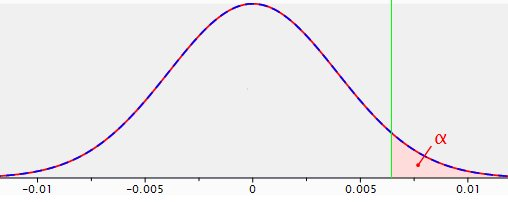This chart reflects what is formally called the ‘sampling distribution for the difference between two proportions.’ It is the probability distribution of all possible sample results calculated for the difference between p1=p2=.04 andn1=n2=5,000. This distribution is the basis –the reference distribution- for null hypothesis testing. The null hypothesis being that there is no difference between the two landing pages. This is the distribution used for actually deciding on significance or non-significance.

p=.04 means 4% conversion. Statisticians usually talk about proportions that can lie between 0 and 1, whereas in the everyday language mostly percentages are communicated. In order to comply with the chart, the proportion notation is used.

This probability distribution can be replicated roughly using this spss syntax (thirty paired samples from a population.sps). Not infinitely, but 30 times two samples are drawn with p1=p2=.04 and n1=n2=5,000. The difference between the two samples are then plotted in a histogram with the normal distribution inputted (the last chart in the output). This normal curve will be quite similar to the curve in figure 1. The reason for performing this experiment is to demonstrate the essence of a sampling distribution.

The modal value of the difference in conversion between the two groups is zero. That makes sense, both groups come from the same population with a conversion of 4%. Deviations from zero both to the left (original does better) and to the right (alternative does better) can and will occur, just by chance. The further from zero, however, the smaller the probability of happening. The pink area with the character alpha indicated in it is the significance area, or unreliability=1-reliability=1-.95.

If in a test the difference in conversion between the alternative page and the original page falls in the pink area, then the null hypothesis that there is no difference between both pages is rejected in favour of the hypothesis that the alternative page returns a higher conversion than the original. The logic behind this is that if the null hypothesis were really true, such result would be a rather ‘rare’ outcome.

The x axis in figure 1 doesn’t display the value of the test statistic (Z in this case) as would usually be the case. For clarity sake the concrete difference in conversion between the two landing pages has been displayed.

So when in a split run test the alternative landing page returns a conversion rate that is 0.645% higher or more than the original landing page (hence falls in the significance area), then the null hypothesis stating there is no difference in conversion between the landing pages is rejected in favour of the hypothesis that the alternative does better (the 0.645% corresponds to a test statistic Z value of 1.65).

Advantage of the approach “default sample size” is that by choosing a fixed sample size, a certain standardization is brought in. Various tests are comparable ‘stand an equal chance’ to that respect.

Disadvantage to this approach is that whereas the chance to reject the null hypothesis when the null hypothesis (H0) is true is well known, namely the self-selected alpha of.05, the chance to not reject H0when H0 is not true remains unknown. These are two false decisions, known as type 1 error and type 2 error respectively.

A type 1 error, or alpha, is made when His rejected, when in fact H0 is true. Alpha is the probability of saying on the outcome of a test there is an effect for the manipulation, while on population level there actually is none. 1-alpha is the chance to accept the null hypothesis when it is true –a correct decision-. This is called reliability.

A type 2 error, or beta, is made when His not rejected, when in fact H0 is not true. Beta is the probability of saying on the outcome of a test there is no effect for the manipulation, while on population level there actually is. 1-beta is the chance to reject the null hypothesis when it is not true–a correct decision-. This is called power.

Power is a function of alpha, sample size and effect (the effect here is the difference in conversion between the two landing pages, i.e. at population level the added value of the alternate site compared to the original site). The smaller alpha, sample size or effect the smaller power is.

In this example alpha is set by the analyst at.05. Sample sizes are also set by the analyst, 5000 for original, 5000 for alternative. Which leaves the effect. And the actual effect is by definition unknown. However it is not unrealistic to use commercial targets or experiential numbers as an anchor value, as was formulated by the marketer in the current case: an expected improvement from 4% to 5%. Now if that effect were really true, the marketer of course would want to find statistically significant results in a test.

An example may help to make this concept insightful and to clarify the importance of power: suppose the actual (=population) conversion of the alternative page is indeed 5%. The sampling distribution for the difference between two proportions with conversion1=4%, conversion2=5% and n1=n2=5,000is plotted in combination with the previously shown sampling distribution for the difference between two proportions with conversion1=conversion2=4% and n1=n2=5,000 (figure 1).

Figure 2: sampling distributions for the difference between two proportions with p1=p2=.04, n1=n2=5,000(red line) and p1=.04, p2=.05, n1=n2=5,000 (dotted blue line), with a one-sided test and a reliability of .95.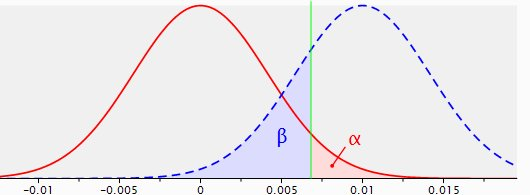The dotted blue line shows the sampling distribution of the difference in conversion rates between original and alternative when in reality (on population level) the original page makes 4% conversion and the alternate page 5%, with samples of 5,000 each. The sampling distribution whenH0 is true, the red line, has basically shifted to the right. The modal value of this new distribution with the supposed effect of 1% is of course 1%, with random deviations both tothe left and to the right.

Now, all outcomes, i.e. test results, on the right side of the green line (marking the significance area) are regarded as significant. All observations on the left side of the green line are regarded as not significant. The area under the ‘blue’ distribution left of the significance line is beta, the chance to not reject H0 when H0is in fact not true (a false decision), and it covers 22% of that distribution.

That makes the area under the blue distribution to the right of the significance line the power area and this area covers 78% of the sampling distribution. The probability to reject H0 when H0is not true, a correct decision.

So the power of this specific test with its specific parameters is .78.

In 78% of the cases when this test is done, it will yield a significant effect and consequent rejecting of H0. Could be acceptable, or could perhaps not be acceptable; that is a question for marketer and analyst to agree upon.

No simple matter, but important. Suppose for example that an expectation of 10% increase in conversion would be realistic as well as commercially interesting: 4.0% original versus 4.4% for the alternative. Then the situation changes as follows.

Figure 3: sampling distributions for the difference between two proportions with p1=p2=.040, n1=n2=5,000 (red line) and p1=.040, p2=.044, n1=n2=5,000 (dotted blue line), with a one-sided test and a reliability of .95.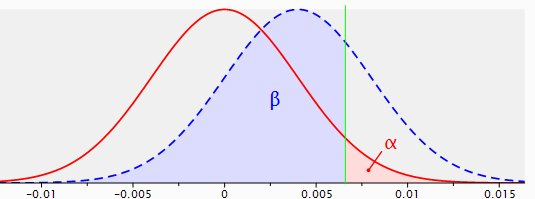Now the power is.26. Under these circumstances the test would not make much sense, is in fact counter-productive, since the chance that such test will lead to a significant result is as low as .26.

The above figures are calculated and made with the application Gpower’:

This program calculates achieved power for many types of tests, based on desired sample size, alpha, and supposed effect.

Likewise required sample size can be calculated from desired power, alpha and expected effect, required alpha can be calculated from desired power, sample size and expected effect and required effect can be calculated from desired power, alpha and sample size.

Should a power of .95 be desired for a supposed p1=.040, p2=.044, then the required sample sizes are 54.428 each.

Figure 4: sampling distributions for the difference between two proportions with p1=p2=.040 (red line) and p1=.040, p2=.044 (dotted blue line), using a one-sided test, with a reliability of .95 and a power of .95.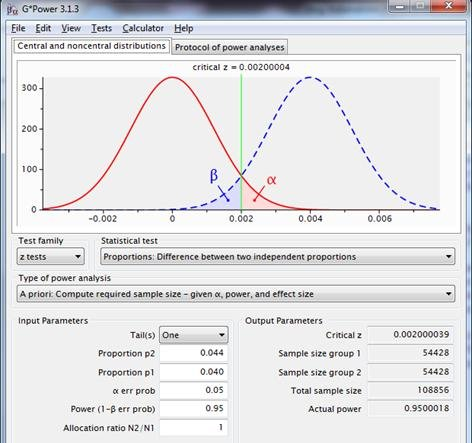This figure shows information omitted in previous charts. This also gives an impression of the interface of the program.

Important aspects of power analysis are careful evaluation of the consequences of rejecting the null hypothesis when the null hypothesis is in fact true – e.g. based on test results a costly campaign is implemented under the assumption that it will be a success and that success doesn’t come true – and the consequences of not rejecting the null hypothesis when the null hypothesis is not true -e.g. based on test results a campaign is not implemented, whereas it would have been a success.

Solution: “default number of conversions”

The analyst says: split run with a minimum of 100 conversions per competing page and a one-sided test with a reliability of .95.

In the current case with expected conversion of the original page 4% and expected conversion of the alternate page 5%, a minimum of 2,500 observations per page will be advised.

When put to the power test though, this scenario demonstrates a power of just little over .5.

Figure 5: sampling distributions for the difference between two proportions with p1=p2=.04, n1=n2=2500 (red line) and p1=.04, p2=.05, n1=n2=2500 (dotted blue line) rusing a one-sided test, with a reliability of .95.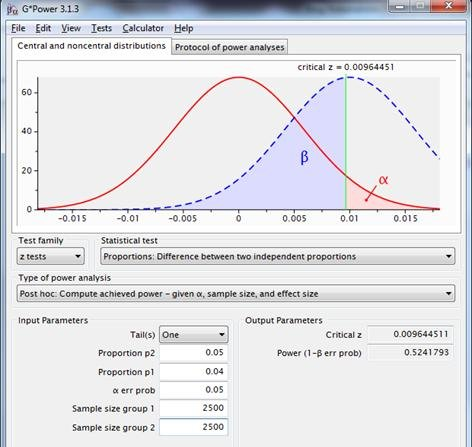For a better power, a greater effect should be present, a larger sample size must be chosen, or alpha should be increased, e.g. to .2:An alpha of .2 returns a power of .8. The power is more acceptable; the ‘cost ‘ for this bigger power consists of a magnified chance to reject H0 when His actually true.

Again, business considerations involving the impact of alpha and beta play a key role in such decisions.

Approach “default number of conversions” with its rule of thumb on the number of conversions actually puts a kind of limit on effect sizes that still make sense to be put to a test (i.e. with a reasonable power). In that regard it also comprises a sort of standardization and that in itself is not a problem, as long as its consequences are understood and recognised.

Solution: “significant sample result”

The analyst says: split run with enough observations to get a statistical significant result if in the test the supposed effect andactually occurs, tested one-sided with a reliability of .95.

That sounds a little weird, and it is. Unfortunately this logic is often applied in practice. The required sample size is basically calculated assuming the supposed effect to actually occur in the sample.

In the used example: if in a test the original has a conversion of 4% and he alternative 5%, then 2,800 cases per group would be necessary to reach statistical significance. This can be demonstrated with the accompanying spss syntax (limit at significant test result.sps).

These sort of calculations are applied by various online tools offering to calculate sample size. This approach ignores the concept of random sampling error, thus ignoring the essence of inferential statistics and null hypothesis testing. In practice, this will always yield a power of .5 plus a small additional excess.

Figure7: sampling distributions for the difference between two proportions with p1=p2=.04, n1=n2=2800 (red line) and p1=.04, p2=.05, n1=n2=2800 (dotted blue line), using a one-sided test, with a reliability of .95.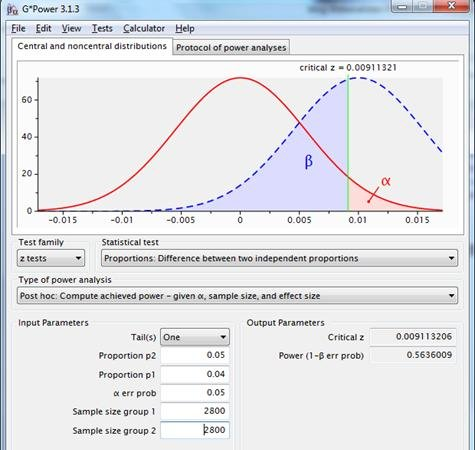Using this system a sort of standardisation is actually also applied, namely on power, but that’s not the apparent goal this method was invented for.

Solution: “default reliability and power”

The analyst says: split run with a power of .8 and a reliability of .95 with a one-sided test.

In the current case with 4% conversion for original page versus 5% expected conversion for the alternate page, alpha=.05 and power=.80, Gpower advises two samples of 5313.

Figure 8: sampling distributions for the difference between two proportions with p1=p2=.04(red line) and p1=.04, p2=.05 (dotted blue line), using a one-sided test with reliability .95 and power .80.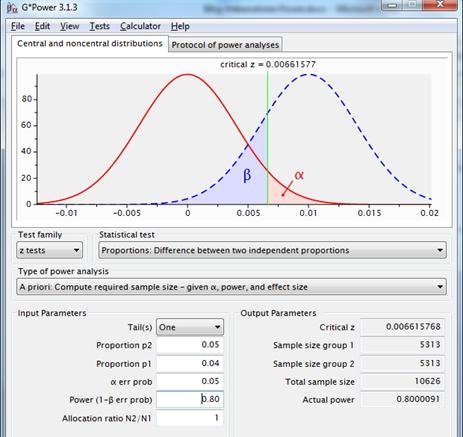This approach uses desired reliability, expected effect and desired power in the calculation of the required sample size.

Now the analyst has grip on the probability an expected/desired/necessary effect will lead to statistically significant results in a test, namely .8.

Some online tools, for example Visual Website Optimizer’s Split Test Duration Calculator, use the concept of power in their sample size calculation.

In a presentation by Visual Website Optimiser “Visitors needed for A/B testing” a power of .8 is mentioned as a regular measure.

It can be questioned why that should be an acceptable rule? Why could the size of the power, as well as the size of the reliability not be used more dynamically?

Solution: “desired reliability and power”

The analyst says: split run with desired power and reliability using a one-sided test.

Follows a discussion on what is acceptable power and reliability in this case, with as a conclusion, say, both 90%. Result according to Gpower, 2 times 5.645 observations:

Figure 9: sampling distributions for the difference between two proportions with p1=p2=.04 (red line) and p1=.04, p2=.05 (dotted blue line), using a one-sided test with reliability=.90 and power=.90.What if the marketer says “It takes too long to gather that many observations. The landing page will then not be important anymore. There is room for a total of 3,000 test observations. Reliability is equally important as power. The test should preferably be carried out and a decision should follow”?

Result on the basis of this constraint: reliability and power both .75. If this doesn’t pose problems for those concerned, the test may continue on the basis of alpha=.25 and power=.75.

Figure 10: sampling distributions for the difference between two proportions with p1=p2=.04, n1=n2=1500 (red line), and p1=.04, p2=.05, n1=n2=1500(dotted blue line), using a one-sided test with equal reliability and power.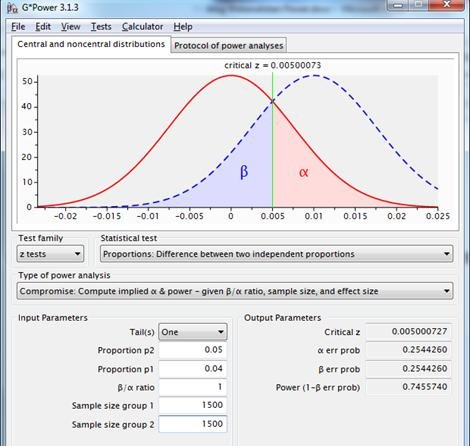This approach allows for flexible choice of reliability and power. The consequent lack of standardization is a disadvantage.

Conclusion

There are multiple approaches to calculate the required sample size, from questionable logic to strongly substantiated.

For strategically important ‘crucial experiments’, preference goes out to the most comprehensive method in which both “desired reliability and power” are involved in the calculation. If there is no possibility of checking against prior effects, an effect can be estimated using a pilot with “default sample size” or “default number of conversions”.

For the majority of decisions throughout the year “default reliability and power” is recommended, for reasons of comparability between tests.

Working with the recommended approaches based on calculated risk will lead to valuable optimization and correct decision making.## Comments (20)

1.Aurelien

Guys it’s way to complicated ! No one of your customers is gonna read that.

You should give an easy rule of thumb to assess if a sample size is big enough or not.

I ran a test and there was like 3 conversions out of 300+ visitors on one side vs. 7 out of 300 conversions on the other side and it says we found a winner. I was surprised because I thought we need about 30 conversions on each side to have definitive results.

Am I wrong ?

2.Siddharth Deswal

Aurelien,

You can use our super simple calculator to see if your result is statistically significant or not. It’s at https://vwo.com/ab-split-significance-calculator/

3.Aurelien

Thank you. The calculator says that I need to have at least 15 conversions for the test to be significant, so how come on the dashboard it says that a winner was found ?

4.Benoit

Except for figure 1, why do the figures caption say “p1=p2=.04 (red line) and p1=.04, p2=.05 (dotted blue line)”?

Shouldn’t it say “p1=.04 (red line) and p2=.05 (dotted blue line)”?

Nice article in any case, glad you shared Gpower.

5.Kees Schippers

Hi Aurelien,

As a proper rule of thumb on required sample size for a desired test, I would recommend
https://vwo.com/ab-split-test-duration/
because they will give a statistical sound advice.

Deciding on significance once you have your results, as in your example, so after the test has been completed, is another thing. Certainly related, but another part of the story.

To explain the background of the article: its intend is to describe the practical consequences of applying currently used rules of thumb on choosing sample size – and sometimes going beyond these rules – in a technical way that will allow for theoretical and empirical verification. If not open for verification or falcification I might just say anything. And that unfortunately is just the problem in the everyday life of marketers depending on analysts advices which cannot easily be evaluated on correctness. Some of these advices are indeed not very usefull all of the time and one in particular is quite erroneous and will lead to costly mistakes when used.

It certainly is no simple matter, I fully agree, but it also is a very important issue in our attempts to learn from our efforts and to make professional and commercial progress.

6.Kees Schippers

Hi Benoit,

Thanks for the compliment!

It is important to realize that with all figures each line represents the difference between two sampling results.

So for instance in figure 2 the blue line has an average of 1%, precisely the expected difference between a sample from a population with a conversion of 4% and a sample from a population with a conversion of 5%. Due to chance there will be deviations both below and above this 1%.

Imagine to take a sample of 5.000 from the first population with conversion 4% and a sample of 5.000 from the second population with conversion 5% and then subtract from the conversion result of the second sample the conversion result of the the first sample. That is actually the test result.

Do that infinitely and you will get the blue line.

Same goes for the red line, except that now population two has the same conversion as population one, namely 4%.

Does this help?

7.Benoit

Ok! So you’re simply noting it with the reference point (p1 for the blue line), to imply that it was centered at zero (p1). Makes sense, thanks for the explanation.

8.Kees Schippers

By the way..should anyone want to send me an e-mail on the matter, I’m abroad at the moment and will read my mail next week, or you can reach me at cornelisremmert@gmail.com.

9.Sami Rageb

Very cool article.

While many people may find this overwhelming and want things simple, it’s always important to have some understanding of the landscape of ‘what you don’t know’, especially if you are intending to make business decisions off of the data.

Please continue to make posts that get into the details, because while many people want things ‘simple’, there are also those of us that want the truth, because when dealing with data nothing is ever simple.

10.Andrew Garibay

I thoroughly enjoy articles like this that go into the details and guide a user through how to assess the validity of their data.

Keep up the good work – I love seeing when your team has pushed a new article live through my reader :]

1.Wingify

@Sami and @Andrew: thanks so much for appreciating the article guys. Kees has written an excellent piece and we’re very proud to host it.

11.Kees Schippers

@Sami and @Andrew, thanks so much for your supportive words. Your appreciation is really stimulating for further writings.

Also, don’t hesitate if you want to propose new topics you would find interesting.

12.Sami Rageb

I’m not sure this is a focus of this blog, but I would like to know more about how A/B/MV testing can be correlated to virality, and perhaps some solid math behind how virality can be calculated.

I’ve seen a range of suggested methods and it’s something that’s often mentioned, but I believe perhaps oversimplified and/or misunderstood.

Thanks!

13.Kees Schippers

@Sami, thanks for the suggestion, I’m afraid I have no ready ideas on this matter now; I might get back on it later.

14.Marius

Hi Kees,

great article. Thanks a lot!
I was wondering though, how to employ this method of precaclulating required sample sizes for tests where the key metric isn’t conversion rate, but revenue per visitor (RPV).

To my understanding, it would be fine to use a t-test on the difference between two independent means (two group). Do you agree? If not, could you provide a sample of how you would approach it?

From my observations, the usually rather large standard deviation (compared to the mean) of RPV tests generates the requirement for huge sample sizes in order to get power up to an acceptable level.

Many thanks!

15.Kees Schippers

Hi Marius,

Thanks for your feedback. I agree with you that a t-test for the difference between two independent means should be appropriate in this case and I share your observation that due to large standard deviations, required sample sizes can be quite large.

It is therefore that I advise to seriously think through the desired alpha. Not for going easy on significance, but for optimized decision making.

Apart from that, I’d afterwards also want to analyse differences in response percentages and differences in the average value amongst the respondents, for further exploration of the effects of the test variant(s).

Regards, Kees

16.Euge

Hi guys,

I agree that the article is way too complicated 😉 also, please change the backgound on this page https://vwo.com/ab-split-test-significance-calculator/ It’s killing!

17.Maitrey Verma

The article is certainly informative. It ventures in great depth and I feel a better way to present this amount of information which is easier to absorb will make it even more useful.

18.Maitrey Verma

Beautiful article. After going through it multiple times, the concept sinks in and has created a strong understanding. This is a must-read article to do A/B Testing.

19.Josh Mckenize

I’m gratified with the way that vwo.com deals with this kind of subject. Usually on point, sometimes controversial, without fail well-researched as well as challenging.

## Revenue Tracking for A/B testing: exciting new feature in Visual Website Optimizer

We are extremely proud to release a brand new feature in Visual Website Optimizer: Revenue…

Read MoreParas Chopra

4 Min Read

## 5 Ways Website Heat Maps and Visual Analytics Help You Read Visitors’ Minds

Before we talk about website heatmaps, let’s talk about people. There are two kinds in…

Read MoreSharan Suresh

9 Min Read

## The Definitive Guide To Optimize Your Conversion Funnel With Push Notifications

Your Conversion Funnel is simply an elaborate roadmap that you create for your potential customers. It…

Read MoreRadhika Chugh

12 Min Read

#### Join 10,000+ Marketing, Product & UX Folks

Latest news, views & inspiration in experience optimization for driving growth

A value for this field is required.